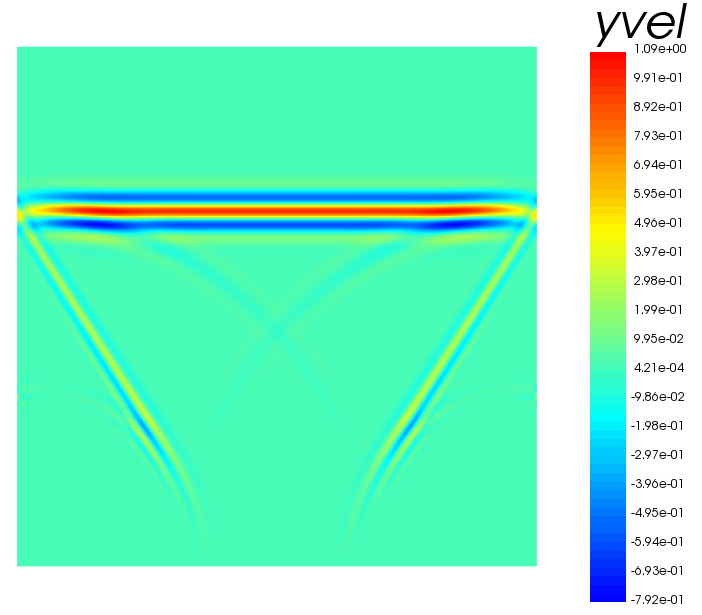# Wave Propagation in 11 Lines of CodeOur Analyst mode offers flexibility in both model setup and simulation. It offers a wide array of functions that can also be used in Review, it can seem a bit daunting given the number of commands. Most of out example models found here normally exceed 100 lines of code however they make use of the common commands needed to set up a model whether the model be mechanical or electro-mechanical you will always find the same commands used respectively for each type.

We have provided the file have a look and see if you can edit it to use less lines of code, or have a go and see what types of models you can make using as least amount of code as possible.

We have created a model that simulates wave propagation in steel in 11 lines of code using only 10 commands and 7 sub-commands, we will walk you through each of the commands as they appear in the model input file:

GRID - The GRID command is used to define the number of nodes that make up the computational grid, the number of dimensions for the model (this example is 2D) and the appropriate constraint relations. Normally you would define your model dimensions and use the dimensions to establish keypoints in this example though we have simply created a model that is made up of 400 nodes in the x and y direction making this a 2D model. Adding another integer value would create a 3D gird.

GEOM - The GEOM command is used to generate a Cartesian mesh within an IJK-region of the mesh. Similarly to how grid works normally this would be defined using keypoints. In this example we have used the sub-commands XCRD and YCRD this used to define grid discretization along the x-axis and y-axis for standard partition nodes. Essentially what this command combined with the GRID command is doing is creating a square region that is 0.1m in x and y with 400 nodes in each direction so we are working with an element size of 250um.

MATR - This command is used to define material properties for each continuum element in the model. We have a selection of materials that are accessible in our material database they can be easily edited giving greater flexibility to the user.

SITE -  This command is used to define the model by assigning material properties to each continuum element of the grid. Material properties are manually defined by the user, which is what is done in this example.

FUNC - The FUNC command allows you to define the characteristics of a special purpose function. Currently available are 7 functions e.g. Ricker Wavelet and Sinusodial Pulse. There is also the option to load in a user defined time function from some external data. Here we are using a Ricker Wavelet function centred at a frequency of 1MHz with an amplitude value of 1.

BOUN - This allows you to assign boundary conditions to the grid boundary surfaces. The Ricker Wavelet input function defined can be called and used throught the script by simply calling 'func'. So here what we are doing when we are using the BOUN command is applying our time function to that side and loading the model on ymin. Normally the BOUN command is used to define boundary conditions such as symmetrical, absorbing, fixed or free. Onscale has a wide range of boundary conditions and they can be freely defined to a region of the model within our Analyst and Designer modes.

PRCS - The PRCS command is required in every model. It checks that your code is correctly set up, initializes the arrays that will hold all the data calculated during execution and most importantly it calculates the timestep required to run the job based on your mesh and materials. Without it, you cannot run the model. The EXEC command would use the time-step determined by PRCS so it will return an error if there was no PRCS command.

EXEC - EXEC is used to compute the response of the model. Normally you would know how long you want to run your model or you could calculate this yourself. Normally you would want to set up a plotting loop after the PRCS step to visualize how your model is affected by the input, this section of our Analyst Tutorial shows to properly set up a plotting loop.

GRPH - The GRPH command provides interactive graphics display of the computational model and results of an analysis. Also provides for interactive or batch mode generation of PostScript image files. For users familiar with our legacy software PZFlex you can also call the graphics use din Flex by calling TYPE STND graphics (we are not doing this here). The solvers automatically calculate a few data arrays the velocity arrays xvel, yvel and zvel are all automatically calculated, normally we would have to calculate output using the CALC command. IN this example we are using the command GRPH PLOT to plot graphically the array yvel.

TERM - This is used to pause the run process, without this the plot will just flash up quickly and close this holds the graphics open until the process is continued. To continue a process once a term has been reached click Run Local again or input 't' into the console.

More commands and sub-commands information can be found in our local help that is only available via the application.

We have provided the file have a look and see if you can edit it to use less lines of code, or have a go and see what types of models you can make using as the least amount of code as possible.